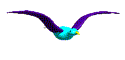Elementary Algebra Assignment #34BSolve the systems for x and y:

 1. 2. 3. 3x + y = 6 x - y = 2 5x + 4y = 1 5x - 7y = 12 -5x - 3y = 3 x + 2y = 5 4. 5. 6. 3x + 2y = -13 2x + 5y = -16  8x + 6y = 10 4x + 6y = 2 4x + 2y = -14 3x - 3y = -24.BACK TO ASSIGNMENTS